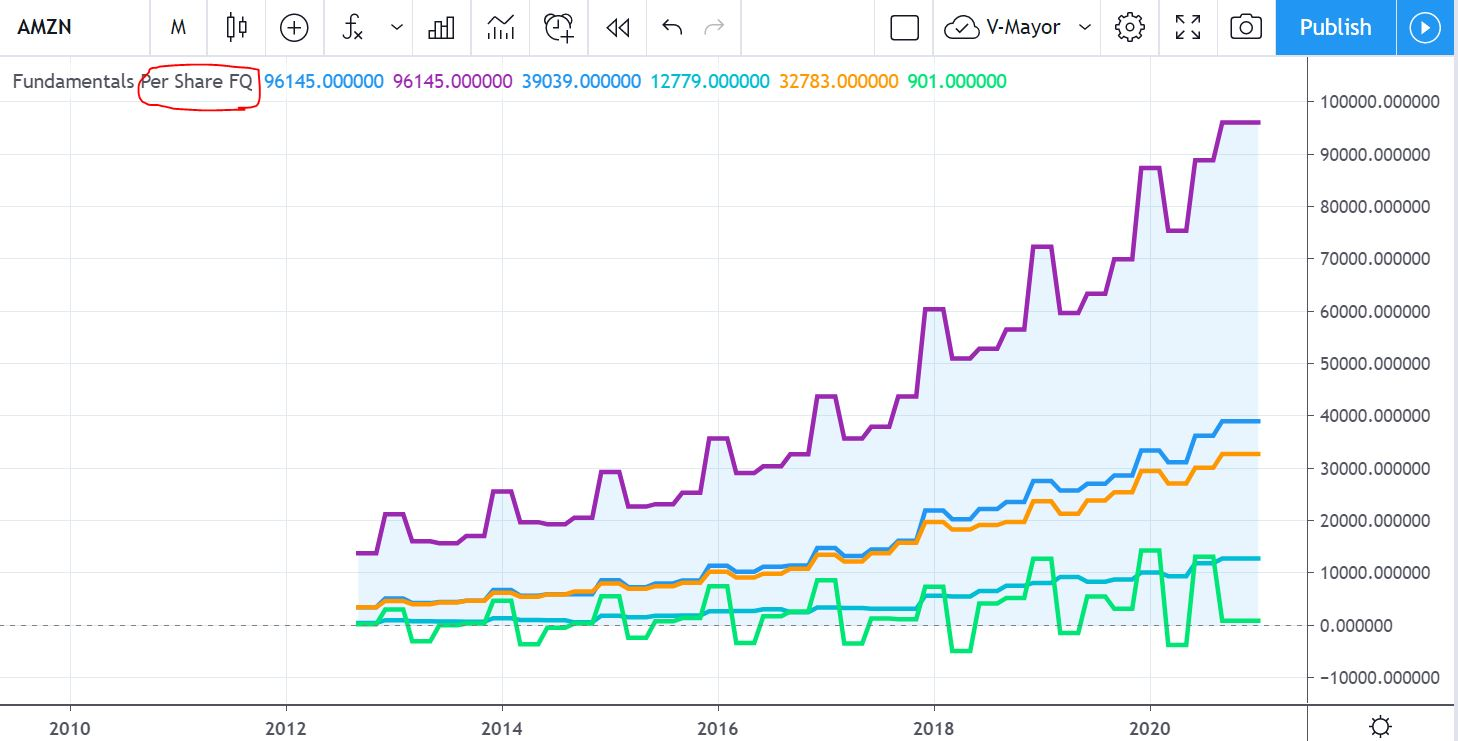This is the first code I have created to show financial information of companies on Tradingview.

To start with something basic I have entered just some data: revenue, gross profit, operating income, EBITDA, and free cashflow.

This link contains the financial data you can use on PINE.

# How it looks like

You can show the data related to the fiscal quarters or the fiscal years (you can do it on configuration).# The code

```// This source code is subject to the terms of the Mozilla Public License 2.0 at https://mozilla.org/MPL/2.0/

//@version=4
study("P1 Fundamentals", shorttitle="Fundamentals", precision=6, overlay=false)
ZEROLINE = 0
MILLION = 1000000

// ---------- Inputs
output = input(defval="Per Share", title='Output type', options=["Per Share", "% of mcap", "Actual"])
// select data by fiscal quarter or by fiscal year
period = input(defval="FQ", title='Period', options=["FQ", "FY"])

// ---------- Variable initializations
// In case you want to add more data you can see all parameters available on the link below

// Revenue and Earnings
rev = financial(syminfo.tickerid, "TOTAL_REVENUE", period) / MILLION
op_income = financial(syminfo.tickerid, "OPER_INCOME", period) / MILLION
grossProfit = financial(syminfo.tickerid, "GROSS_PROFIT", period) / MILLION
ebitda = financial(syminfo.tickerid, "EBITDA", period) / MILLION
op_expenses = financial(syminfo.tickerid, "OPERATING_EXPENSES", period) / MILLION * -1
fcf = financial(syminfo.tickerid, "FREE_CASH_FLOW", period) / MILLION

// Show it? //
showR_E = input(true, title = "Show Revenue & Earnings?")
plot(showR_E?rev:na, title="Revenue", color=color.blue, linewidth=3, style=plot.style_area, transp=90)
plot(showR_E?rev:na, title="Revenue", color=color.purple, linewidth=3, style=plot.style_linebr)
plot(showR_E?grossProfit:na, title="Gross Profit", color=color.blue, linewidth=3, style=plot.style_linebr)
plot(showR_E?ebitda:na, title="EBITDA", color=color.aqua, linewidth=3, style=plot.style_linebr)
plot(showR_E?op_expenses:na, title="Operating Expenses", color=color.orange, linewidth=3, style=plot.style_linebr)
plot(showR_E?fcf:na, title="FCF", color=color.lime, linewidth=3, style=plot.style_linebr)
hline(ZEROLINE)```

I will do some other similar ones with: Basic EPS, data related to debt, and some data related to the balance sheet.

Any suggestion of improvement is welcome.